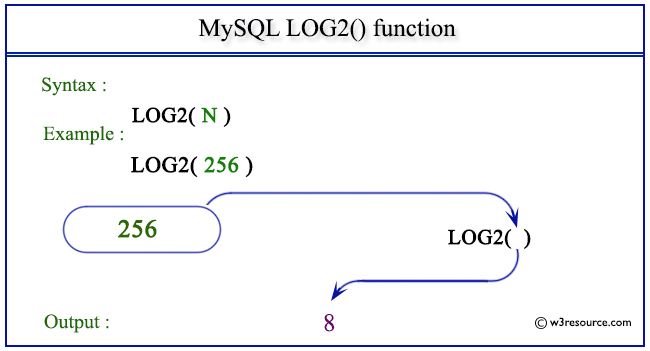# MySQL LOG2() function

## LOG2() function

MySQL LOG2() returns the natural logarithm of a number to the base 2.

Syntax:

```LOG2(N);
```

Argument

Name Description
N A number.

Note :The equivalent expression for this function is LOG(N) / LOG(2).

Syntax Diagram:MySQL Version: 5.6

Pictorial presentation of MySQL LOG2() functionExample of MySQL LOG2() function

Code:

``````SELECT LOG2(256);
```
```

Explanation

The above MySQL statement returns the natural logarithm of 256 as specified in the argument to the base 2.

Sample Output:

```mysql> SELECT LOG2(256);
+-----------+
| LOG2(256) |
+-----------+
|         8 |
+-----------+
1 row in set (0.00 sec)
```

All Mathematical FunctionsPrevious: LOG()
Next: LOG10()

﻿